# Quiz 7: Single Family Housing: Pricing, Investment, and Tax Considerations

a. In order to choose between rent a house or buy a property the computation of IRR (Internal Rate of Return) will be required. Here, the IRR would be the after-tax IRR after considering the tax advantage availed by the person considering to rent a house or to buy a property. Also, the time of selling the property would be considered to make decision. The computation of IRR for the given question is provided below.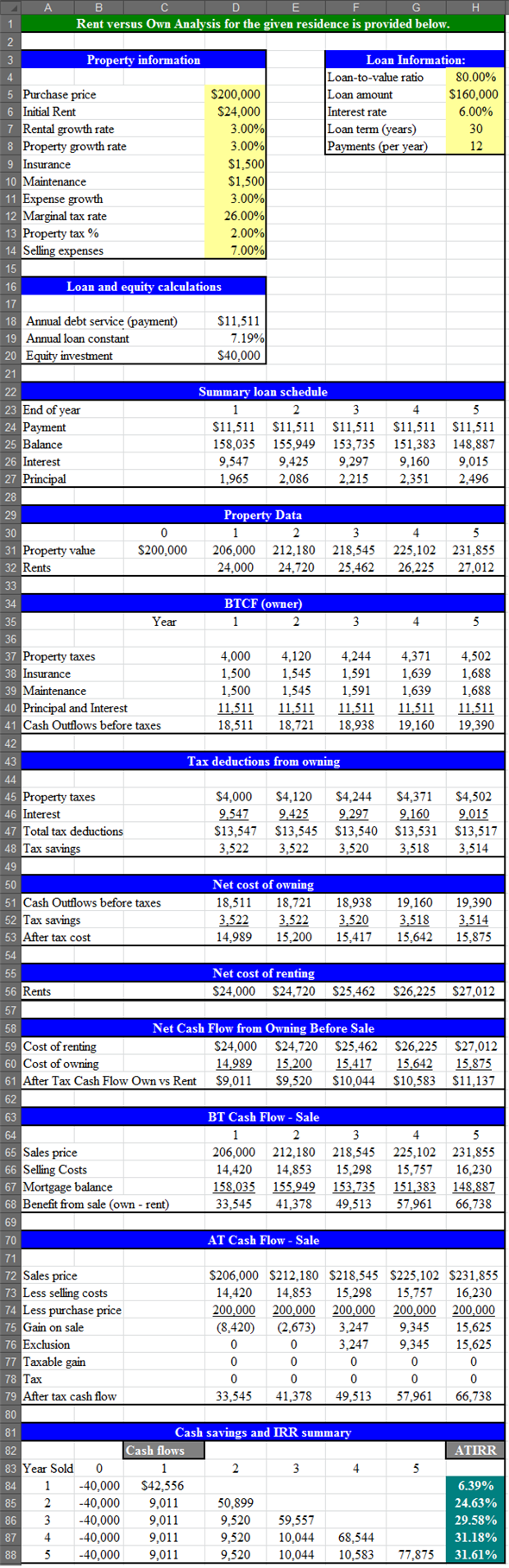Image - A.1 The required IRR is 10%.It is evident from the Image - A.1 that the IRR of the analysis in the year-4 would be 31.18%. Thus, the decision of buying the property would be better. Enter the given values and formulas in the spreadsheet as shown in the image below. In Cells A1:D32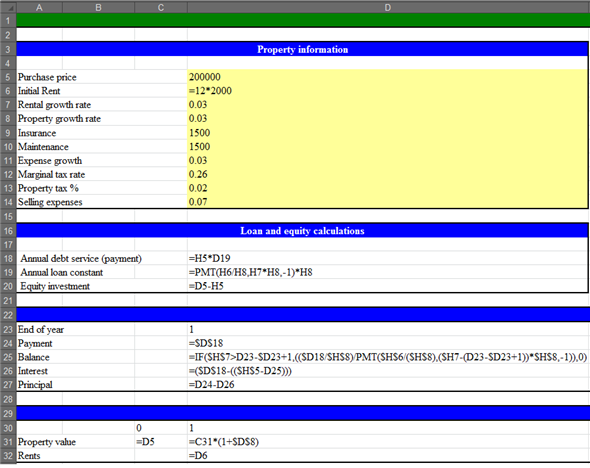In Cells E1:E32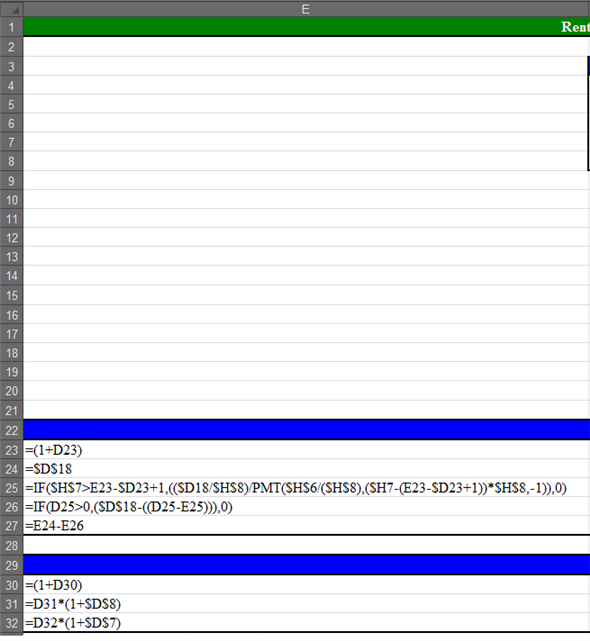In Cells F1:F32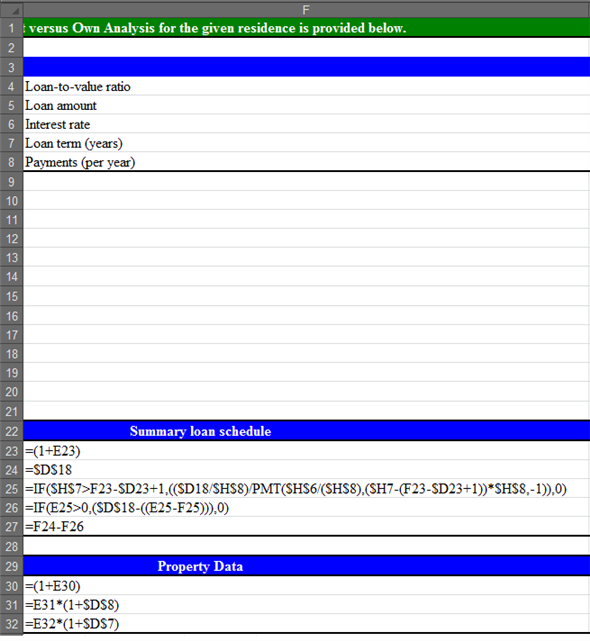In Cells G1:G32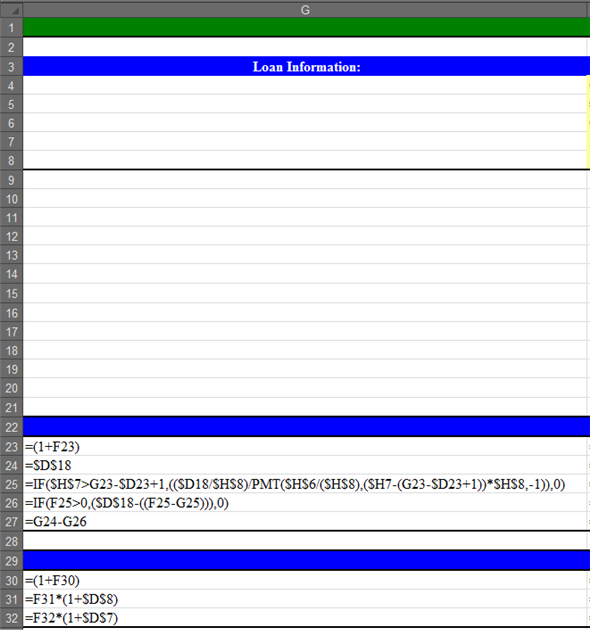In Cells H1:H32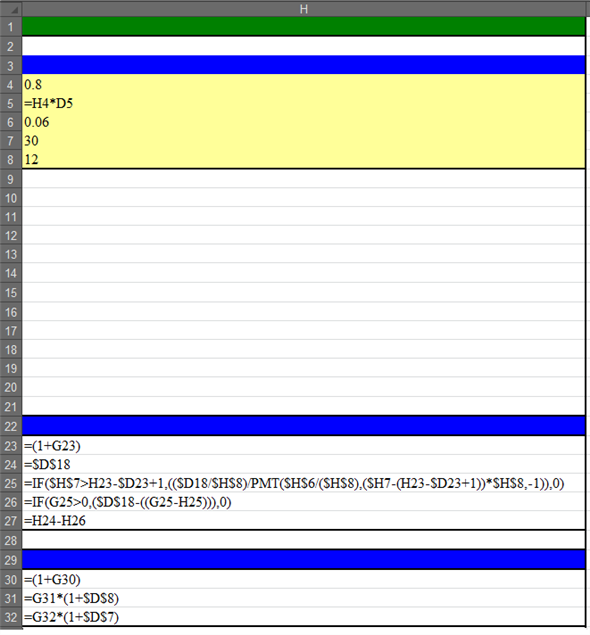In Cells A33:E61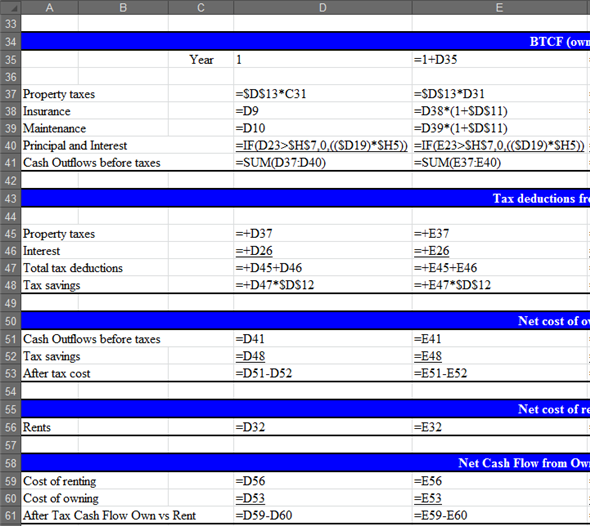In Cells E33:H61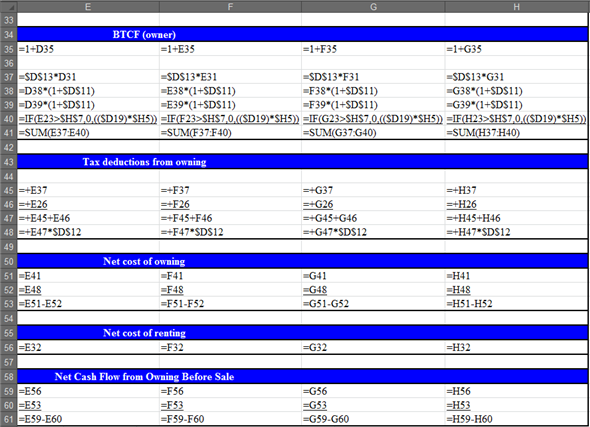In Cells A1:E88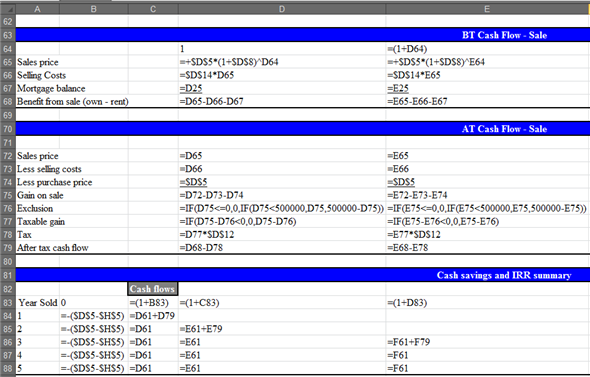In Cells E62:H88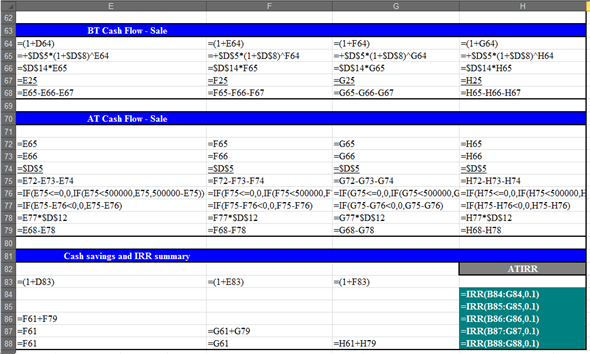b. The required IRR of the person is 10%. It is evident from the Image - A.1 that the ATIRR (After-Tax Internal Rate of Return) in the year-5 is 31.61%. Thus, the owning of the property is better option for the person looking for the property. c. In order to find the level of rent at which will generate the IRR of 4.50% and the investor will be indifferent between buying and renting the property use the "GOAL SEEK" function of spreadsheet. Apply the "GOAL SEEK" on the same spreadsheet as shown in the image below.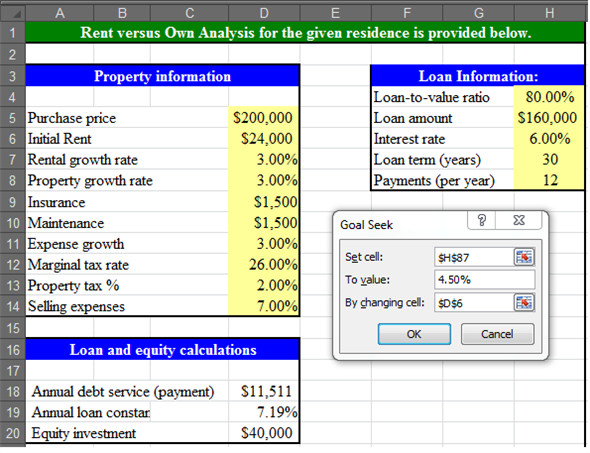The obtained result is provided below.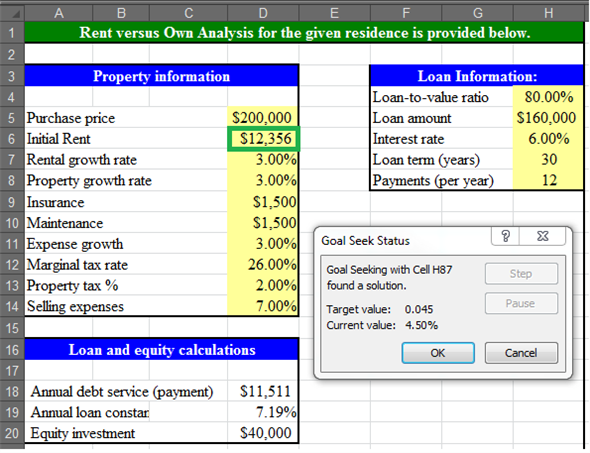Thus, to be indifferent between buying and renting the house the annual rent must be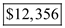. Also, this annual rent will result in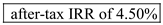.
Return on property Mr. wants to purchase a property today for the value of \$300,000. He wants to finance the property with 80% loan and 20% from his own income. He is expecting that the value of property will increase by 4% annually for next three year. Value of property today = \$300,000 Annual price appreciation rate = 4% Number of year for price appreciation = 3 year Property finance through loan = 80% a. Value of loan is calculated below:Value of loan is calculated below:Value of property after three year with annual appreciation rate of 4percent is calculated below:After three year the value of property will be \$337,459 from which the amount of \$240,000 should be pay for the loan so total amount remains for home equity is calculated below: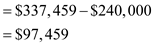Mr. X has invested \$60,000 as home equity and gets a total \$97,459 as return after three year so annual expected rate of return is calculated below:Thus Expected annual average rate of appreciation on home equity will be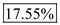. b. Now if value of value will appreciation in uneven rate like in first year at 0%, in second year at 2% and in third year at 3%. So value of property after three year is calculated below:After three year the value of property will be \$315,180 from which the amount of \$240,000 should be pay for the loan so total amount remains for home equity is calculated below: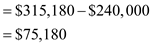Mr. X has invested \$60,000 as home equity and gets a total \$97,459 as return after three year so annual expected rate of return is calculated below:Thus Expected annual average rate of appreciation on home equity will be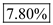.